# Module 5: CONDITIONAL STATEMENTS

## Learning Objectives

After reading this lesson, you should be able to:

• identify different relational operators,

• construct logical expressions,

• perform data type identification with MATLAB functions,

• round up, round down, and round numbers to integers.

## What are conditions?

Conditions are simply logical expressions: they are not unique to programming. You are likely familiar with relational operators like $$\mathbf{<}$$, $$\mathbf{\leq}$$, $$>$$, etc. (although the syntax may be slightly different in MATLAB). This is the basic syntax we use to create conditions in MATLAB. These conditions are either true or false. Either 4 < 5 (four is less than five) or it is not. Note the last two operators seen in Table 1 can also be used with non-numeric values like text. That is, you cannot ask if one word is quantitatively greater than another, but you can ask if they are the same word or not. Note the conditional operator for comparing two values to see if they are equal (==) is not the same as setting a variable equal to a value (=).

Table 1: Relational operators in MATLAB and what they mean.

Logical Query Relational Operator
Is A greater than B? A > B
Is A greater than or equal to B? A >= B
Is A less than B? A < B
Is A less than or equal to B? A <= B
Is A equal to (the same as) B? A == B
Is A not (the same as) B? A ~= B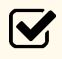Important Note: Beware of “==” and “=”. MATLAB treats them differently, and it will not always warn you of your mistake.

In Example 1, you can see some examples of these relational operators. They return logical values (true or false), which we will discuss in more detail later in this lesson.

### Example 1

Given two variables a and b, conditionally check whether

1. a is less than b,

2. a is equal to b, or

3. a is not equal to b.

You may assume that a and b each store a value that is a real number.

Solution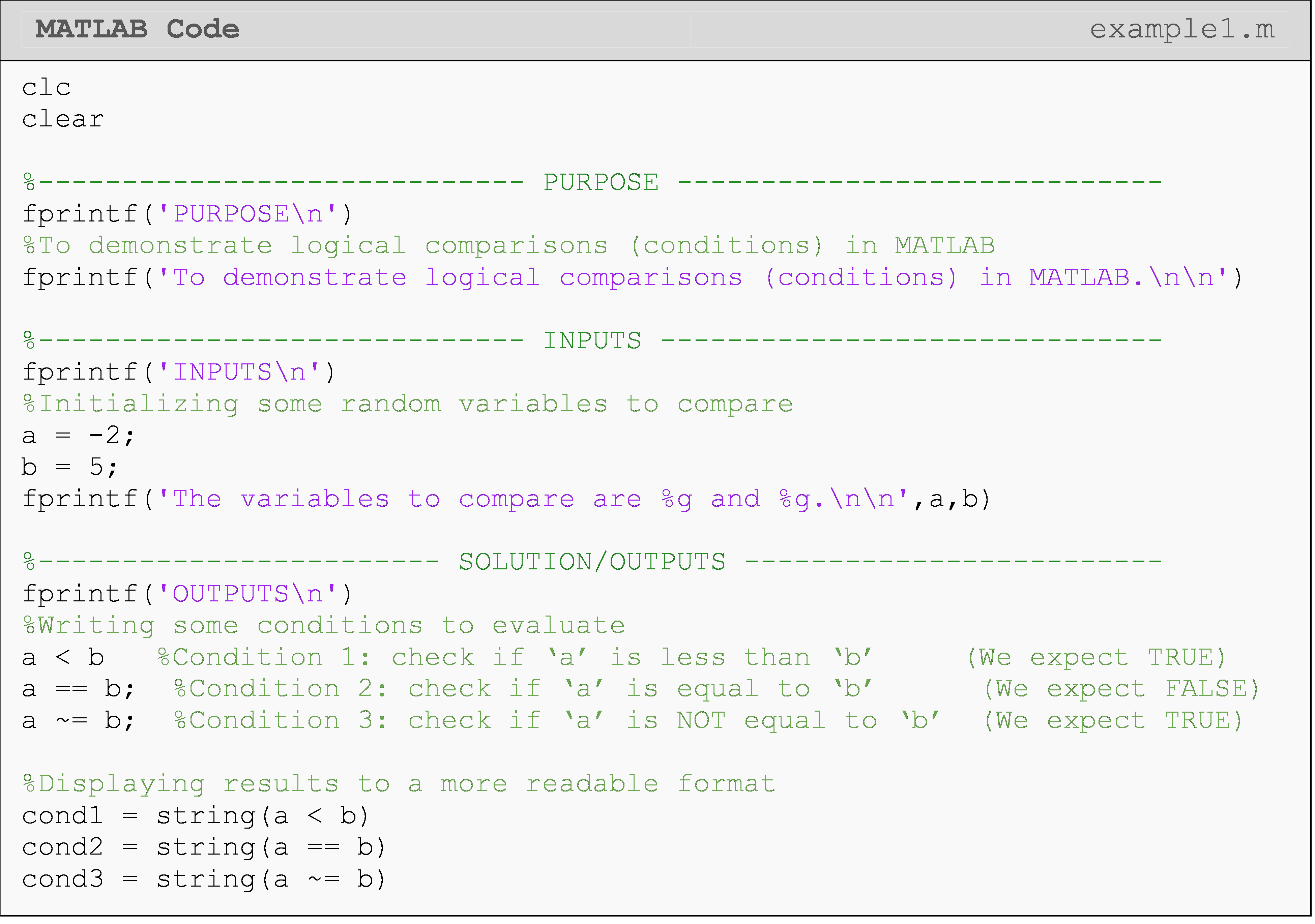In the last part of the solution, we convert the native output of a logical comparison into a more readable format using the MATLAB function string(). Observing the program outputs shown in Command Window reveals that a logical comparison like a < b has a messy output, which includes the tag "logical". To convert this output to something more readable we use string().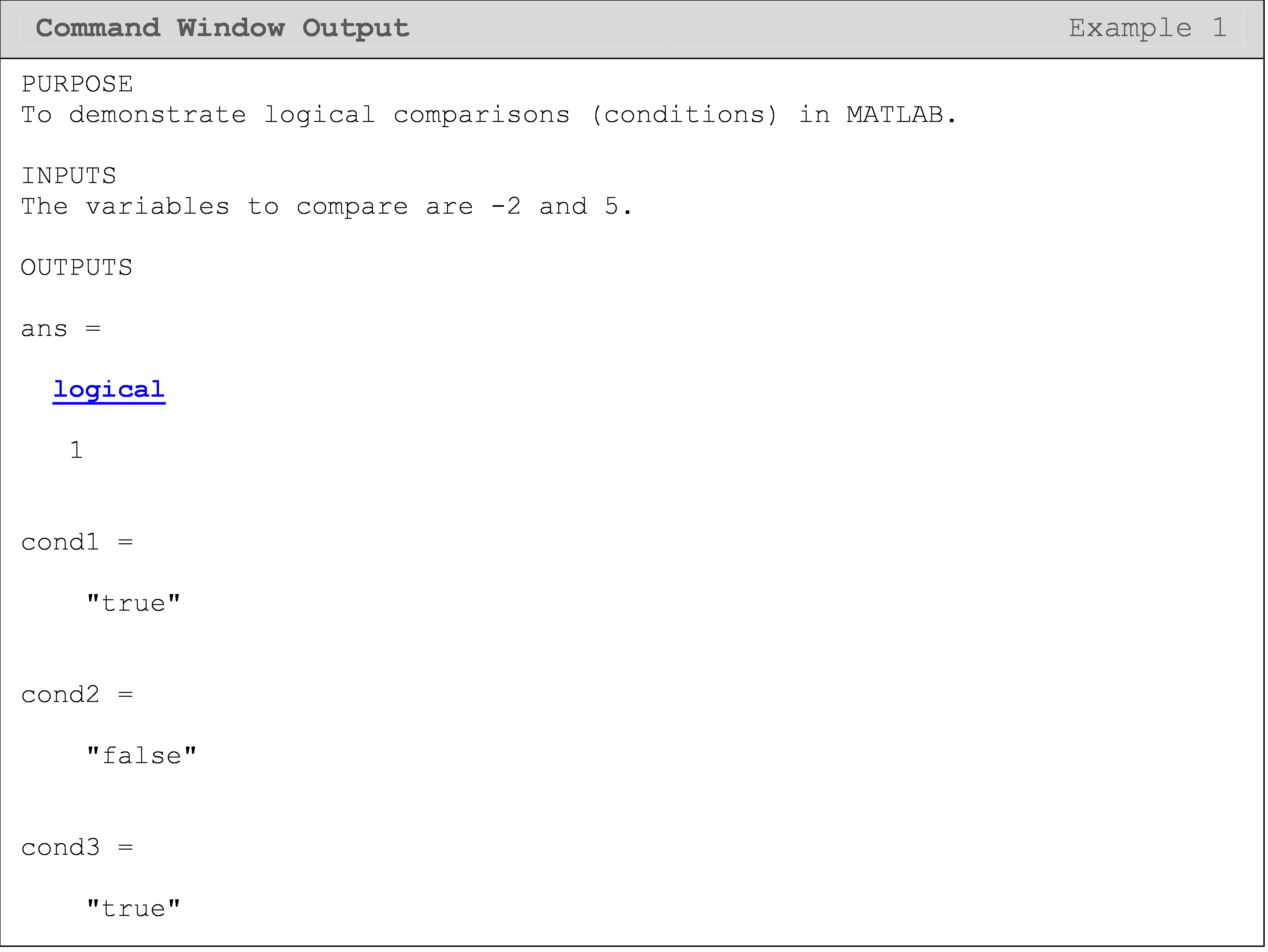## What is Boolean logic?

Boolean values of 1 and 0, or true and false, respectively, represent a new data type in MATLAB called the logical data type. These values are binary (meaning they only have the two possibilities) and will act as such in all cases.

Conditional clauses (expressions that evaluate as true or false like 4 < 5 or 0 == 1) can be stacked together with conditional-linking operators. That is, we can combine these conditional statements. We will cover the two most common condition-linking operators: AND and OR. Just like we use the conjunctions “and” and “or” in speech/language to join independent clauses, we must use them to join two or more conditions together in conditional expressions. Note that in Example 2 the conditional-linking operator is AND (represented by “&&”) and OR (represented by “||”).

When using the && comparison, all logic tests joined by the & must be true for the body of an if-statement to execute. For example, 3>2 && 7>8 would not execute the body of an if-statement. However, when the || comparison is used, only one of the joined tests must be true to execute the body. For instance, 3>2 || 7>8 would execute the body of the if-statement. Finally, (3>2 && 7>8) || 1<=2 would evaluate as true since 1<=2 is true! This is an example of linking Boolean operators together, which is perfectly valid. As a side note, you can use logical() to convert numeric values to the logical data type in MATLAB. This might be especially useful when converting a matrix with numerical values to logical values.

### Example 2

Given the variables, a = 6 and b = 3.4, conditionally check whether a is greater than 1 and less than 5 and whether b is greater than 10 or equal to 3.4.

Solution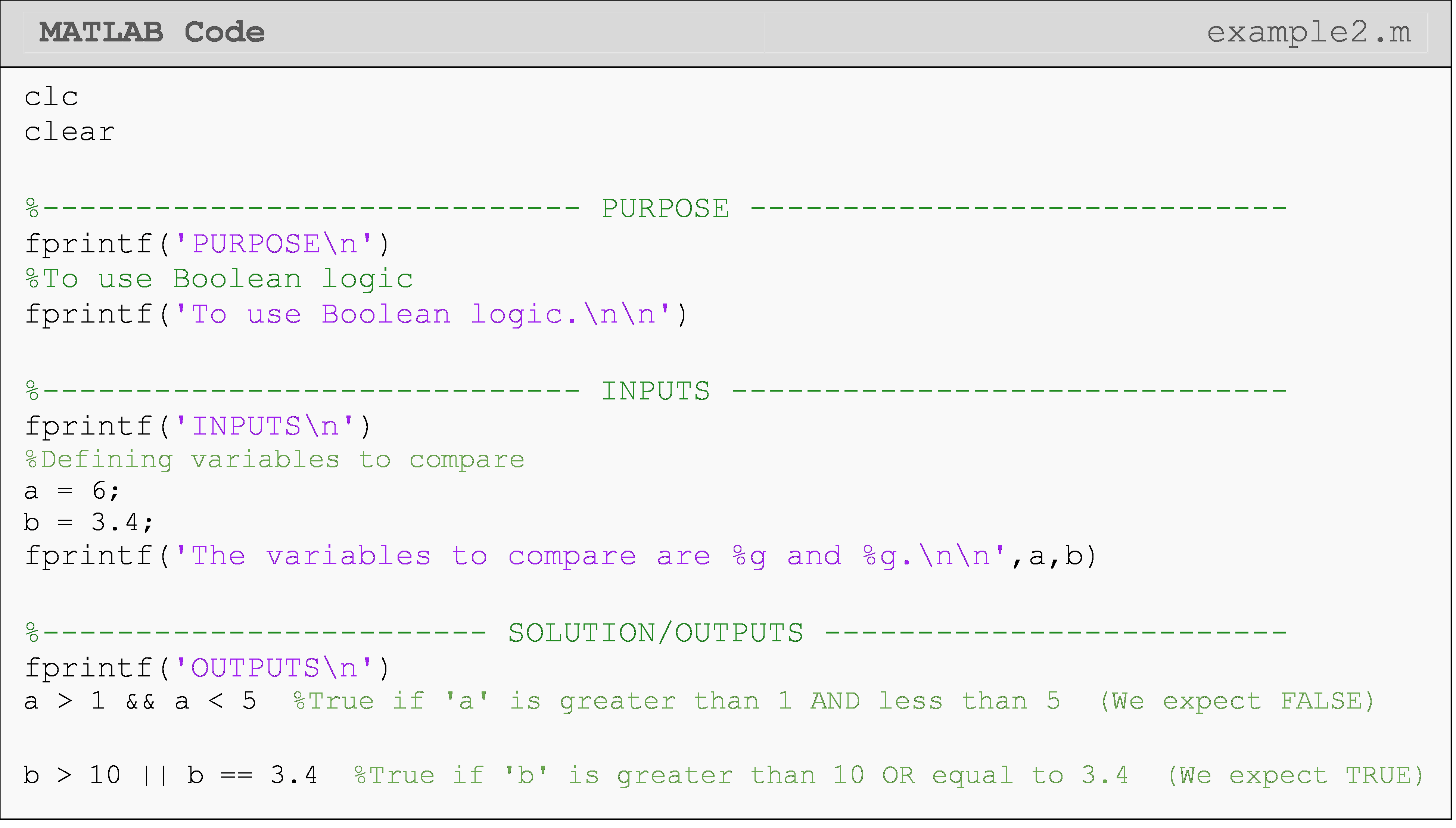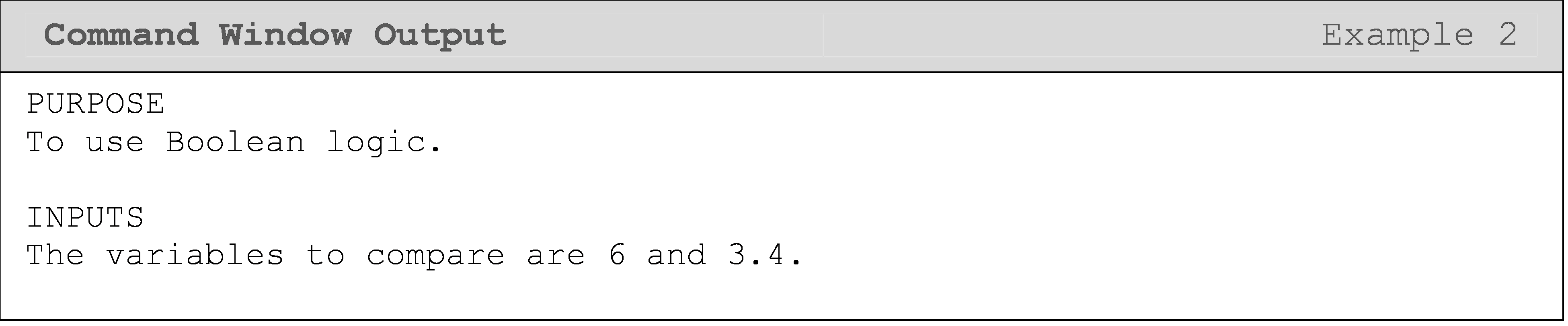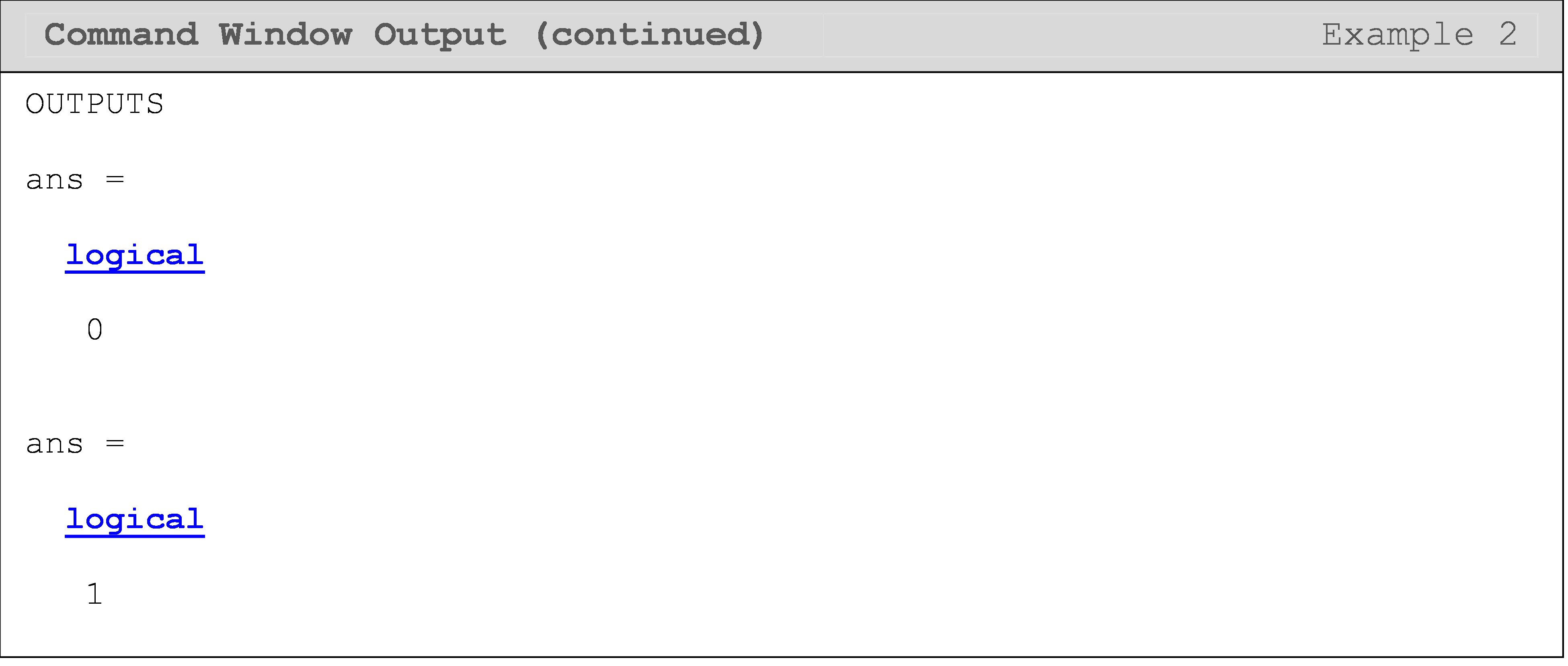## Can different data types be identified in MATLAB?

As you have likely experienced by now, data types must be handled with care. As a result, it can be useful to conditionally check the data type of a variable. MATLAB has handy functions for just such a purpose that return a Boolean value, which has a logical data type, of course. (We covered these previously in Lesson 2.5 (Data Types), and include them again here for clarity.)

These are called the data type identification functions, and some examples include testing whether a number is real or imaginary with isreal() or whether the value of a variable is a character data type with ischar().

### Example 3

Check whether a variable is a char data type or not. Output the class (data type) of the variable to the Command Window.

Solution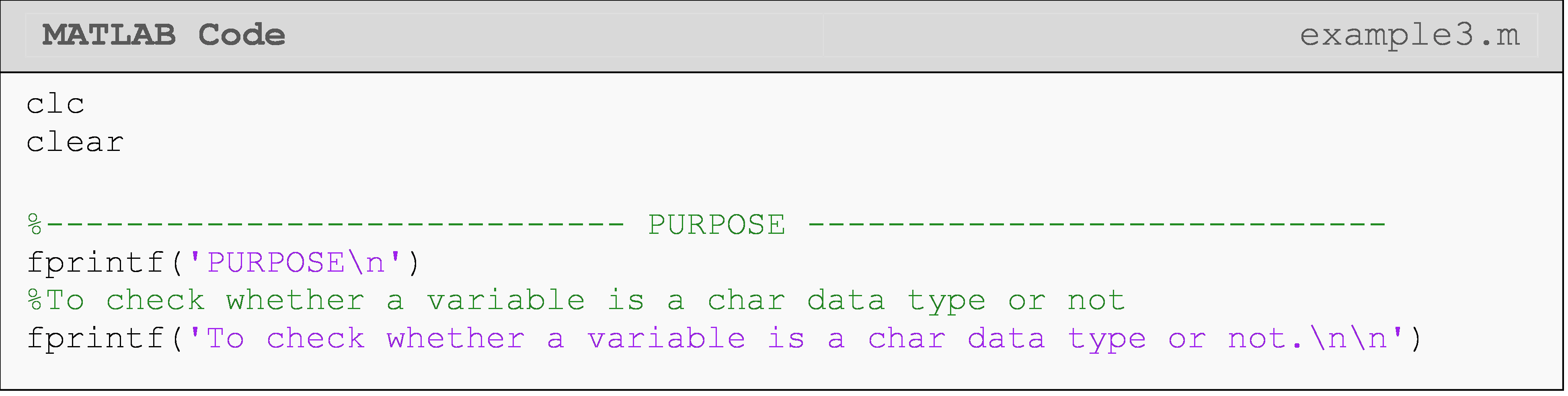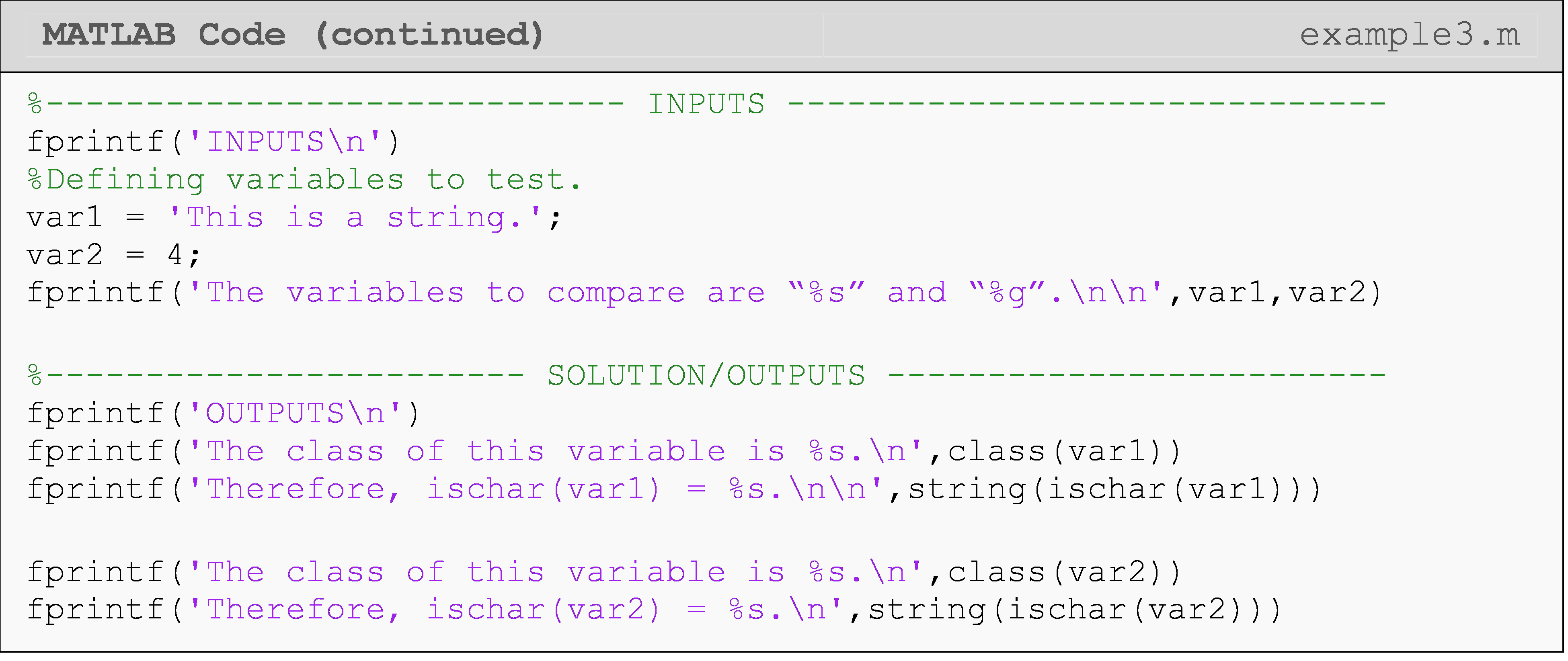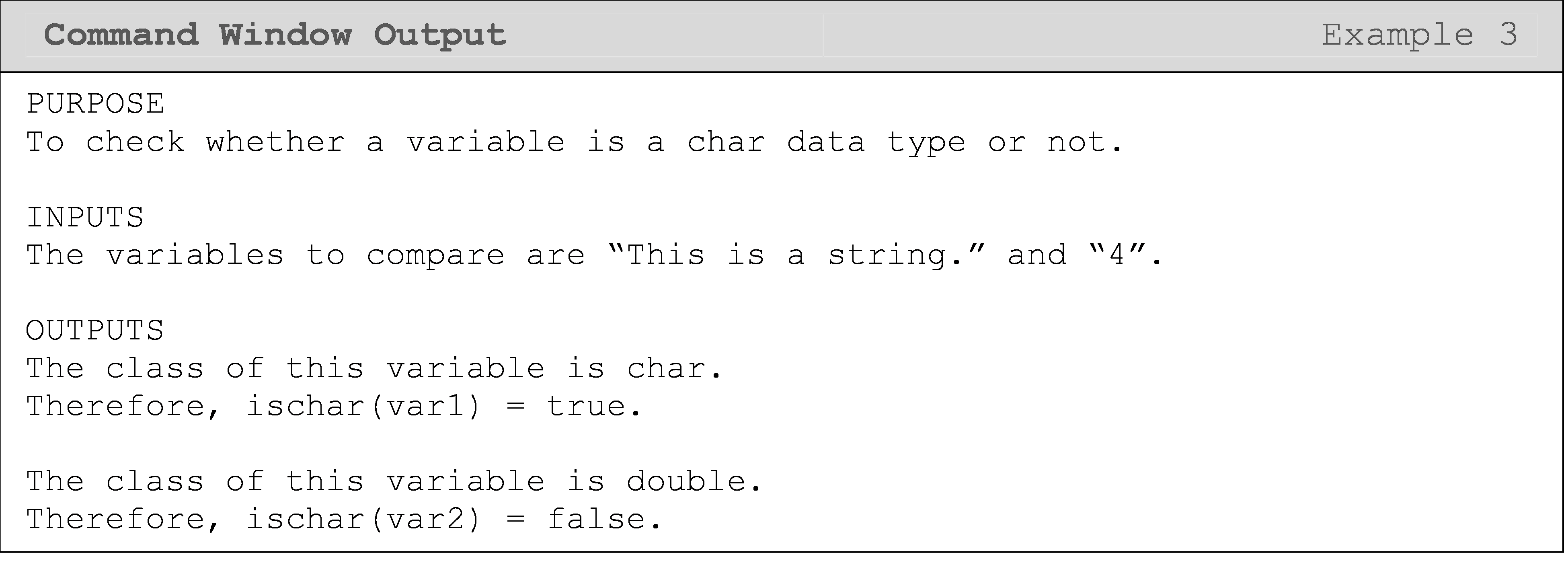## How can I round numbers in MATLAB?

Rounding functions can be very useful in writing effective conditions as we will demonstrate in the following lessons. First, though, we need to know the different rounding functions and how they work. Below is a list of the three most common rounding functions in MATLAB and what they do. You can see each of these functions implemented in MATLAB in Example 4.

• round(): returns the nearest integer (“normal” rounding)

• Example: round(1.5) = 2

• Example: round(1.1) = 1

• ceil(): returns the smallest integer that is greater than or equal to the number

• Example: ceil(1.1) = 2

• Example: ceil(1.7) = 2

• floor(): returns the greatest integer that is less than or equal to the number

• Example: floor(1.3) = 1

• Example: floor(1.9) = 1

### Example 4

Show an example of how the MATLAB functions round(), ceil(), and floor() each round numbers.

Solution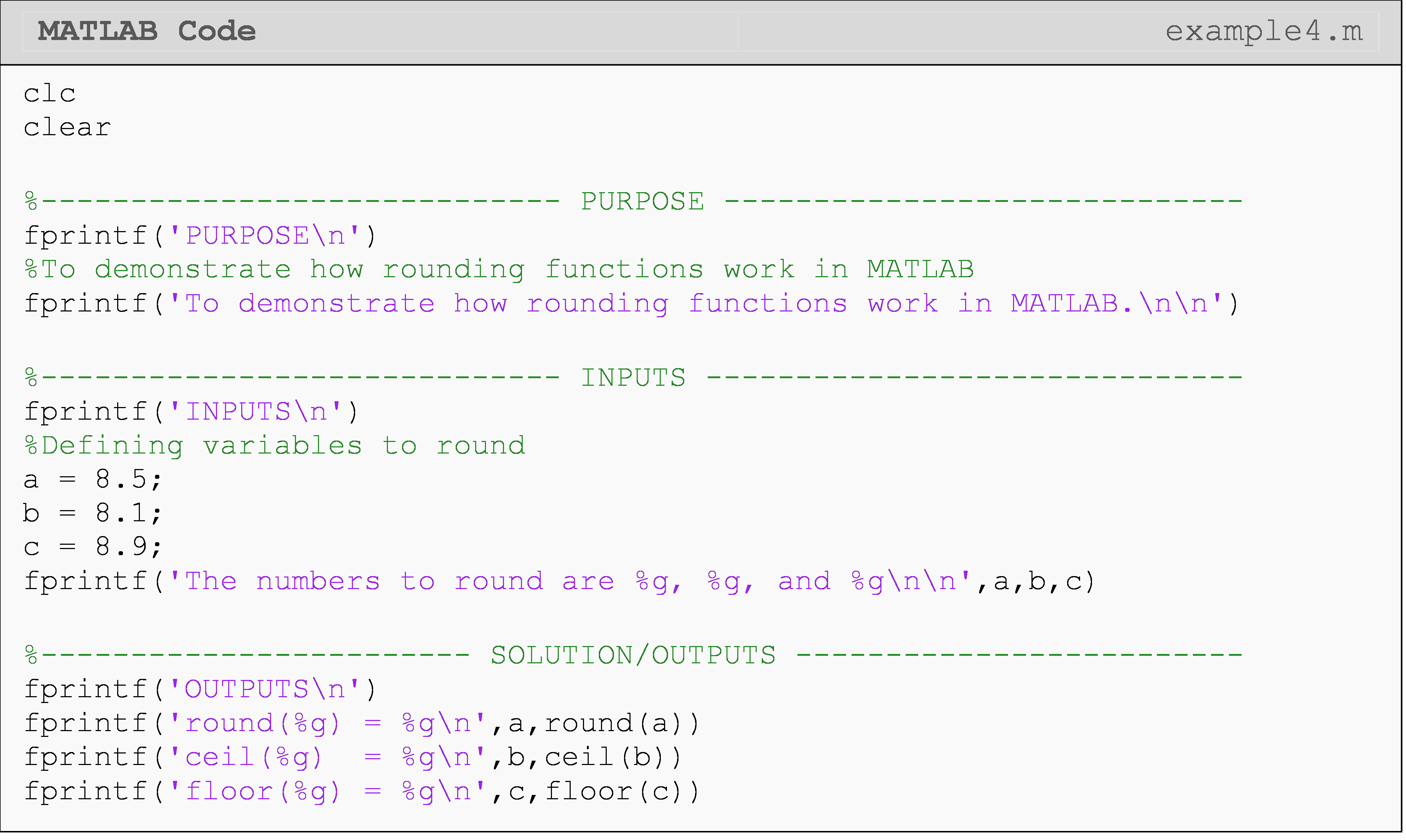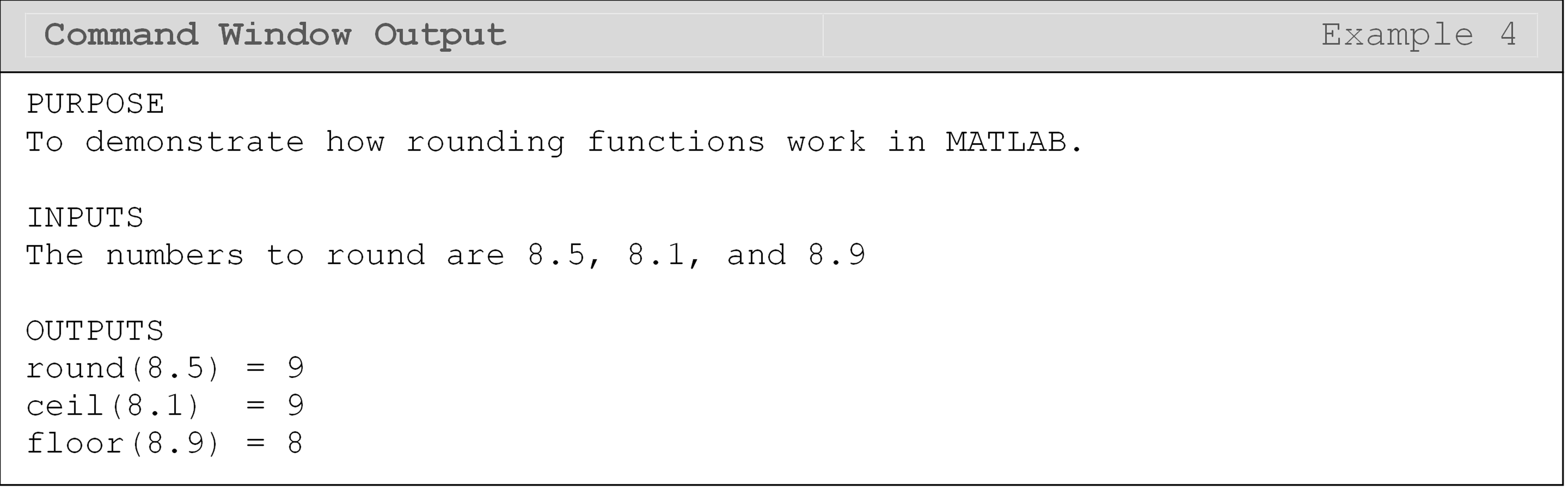## Lesson Summary of New Syntax and Programming Tools

Boolean AND operator && a && b
Boolean OR operator || a || b
Round a number to the nearest integer round() round(a)
Round a number up to the nearest integer ceil() ceil(a)
Round a number down to the nearest integer floor() floor(a)
Check if a variable is a char data type or not ischar() ischar(a)
Check if a variable is a real number or not isreal() isreal(a)
Determine if A is greater than B > A > B
Determine if A is greater than or equal to B >= A >= B
Determine if A is less than B? < A < B
Determine if A is less than or equal to B <= A <= B
Determine if A is equal to (the same as) B == A == B
Determine if A is not (the same as) B ~= A ~= B

## Multiple Choice Quiz

(1). The ~= operator stands for

(a)  approximately equal to

(b)  equal to

(c)  greater than or equal to

(d)  not equal to

(2). sin(pi)==0 gives false as output because

(a)  sin(pi)=1

(b)  sin(pi)=-1

(c)  sin(pi) is not defined

(d)  sin(pi) gives a value other than zero in MATLAB

(3). The operator || stands for

(a)  and

(b)  or

(c)  not

(d)  not equal to

(4). The operator && stands for

(a)  and

(b)  or

(c)  not

(d)  not equal to

(5). What is the Command Window output of the following program?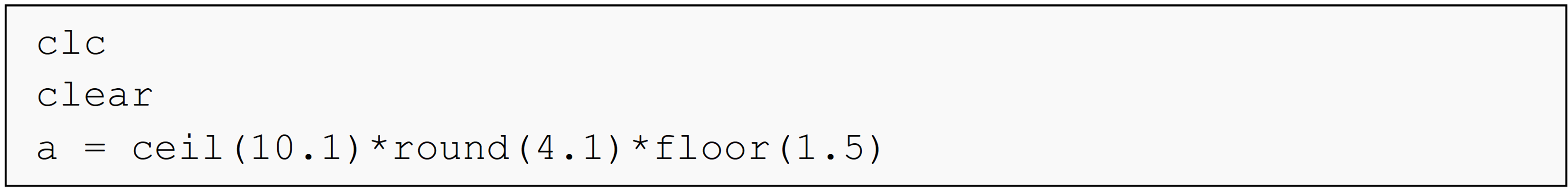(a)  a = 60.6

(b)  a = 44

(c)  a = 60

(d)  a = 66

## Problem Set

(1). Write a condition that evaluates as true when the given variable length is greater than 1.5. Test your condition using length = 1 and then using length = 3.

(2). Write a condition that evaluates as false whenever the given variable age is less than 21. Test your condition using age = 6 and then using age = 30.

(3). Write a condition that evaluates as false when base is equal to 5. Test your condition using a) base = 0.2 and b) base = 5.

(4). Write a set of conditions that evaluates as true when the rounded value of the given variable num is greater than 16 and less than or equal to 21. Test your condition using a) num = -8 and b) num = 17.

(5). Write a set of conditions that evaluates as true when the given variable flag1 is equal to 2 or 3. Test your condition using a) flag1 = 2 and b) flag1 = 0`.

(6). An instructor wants to round up students’ grades to the next integer. Write a program that takes students’ grades as an input and returns the integer grades as an output. Hint: you will need to use a vector as the input/output.